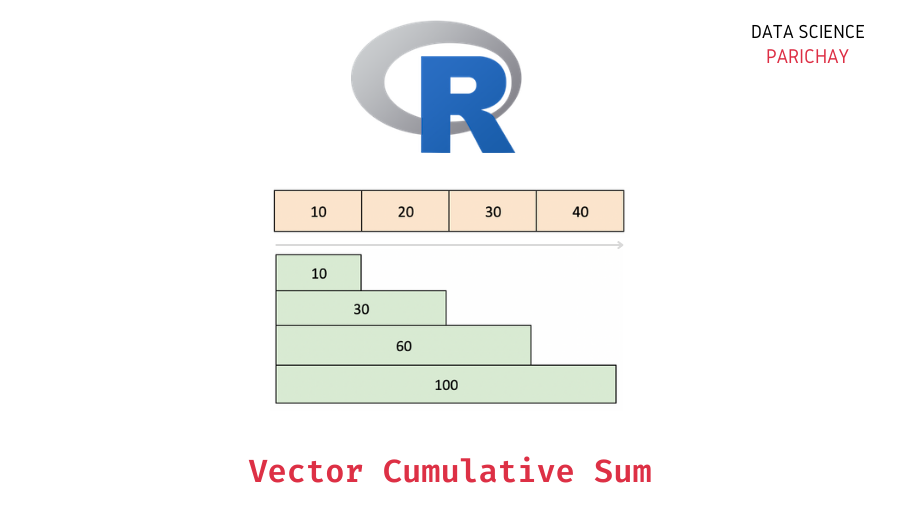# R – Vector Cumulative Sum (With Examples)

Vectors are used to store one-dimensional data of the same type in R. In this tutorial, we will look at how to get the cumulative sum of a vector in R with the help of some examples.

## What is the cumulative sum?

The cumulative sum of a series of values is the sum of values up to that value in our series. For example, for a vector of three values (a1, a2, and a3), the cumulative sum would be a1, a1+a2, and a1+a2+a3. The following image illustrates this with an example.

In the above image, we have four values 10, 20, 30, and 40. The cumulative sum for these values is 10, 10+20, 10+20+30, and 10+20+30+40 respectively. Note that the order in which these values appear is important when computing the cumulative sum.

## How to calculate the cumulative sum of a vector in R?

You can use the `cumsum()` function in R to compute the cumulative sum of the values in a vector. Pass the vector as an argument to the function. The following is the syntax –

```# cumulative sum of vector x
cumsum(x)```

It returns a vector containing the cumulative sum of the values in the passed vector.

## Examples

Let’s now look at some examples of using the above syntax.

### Cumulative sum of a vector of numbers

Let’s create a vector of some numbers and use the `cumsum()` function to calculate its cumulative sum. For example, let’s compute the cumulative sum for the vector `c(10, 20, 30, 40)`.

```# create a vector
vec <- c(10, 20, 30, 40)
# cumulative sum of vector
print(cumsum(vec))```

Output:

📚 Data Science Programs By Skill Level

Introductory

Intermediate ⭐⭐⭐

🔎 Find Data Science Programs 👨‍💻 111,889 already enrolled

Disclaimer: Data Science Parichay is reader supported. When you purchase a course through a link on this site, we may earn a small commission at no additional cost to you. Earned commissions help support this website and its team of writers.

`  10  30  60 100`

We print the resulting cumulative sum. You can see that each value in the cumulative sum vector is the sum of all values till that particular index from the original vector.

### Cumulative sum of a vector with `NA` values

What would happen if you apply the `cumsum()` function to a vector containing some `NA` values?

Let’s find out.

For this, we will create a vector with some `NA` values and then apply the `cumsum()` function.

```# create a vector with NA values
vec <- c(10, 20, NA, 30, NA, 40)
# cumulative sum of vector
print(cumsum(vec))```

Output:

` 10 30 NA NA NA NA`

You can see that we get the cumulative sum till we encounter the first `NA` in our vector. From this point onwards, the resulting cumulative sum for all the values is `NA`. This happens because performing any arithmetic operation with `NA` results in an `NA` in R.

If you want to compute the cumulative sum irrespective of the `NA` values, you can first remove the `NA` values from the vector and then apply the `cumsum()` function.

You might also be interested in –

••